Ex.3.7 (Optional)

Chapter 3 Class 10 Pair of Linear Equations in Two Variables
Serial order wise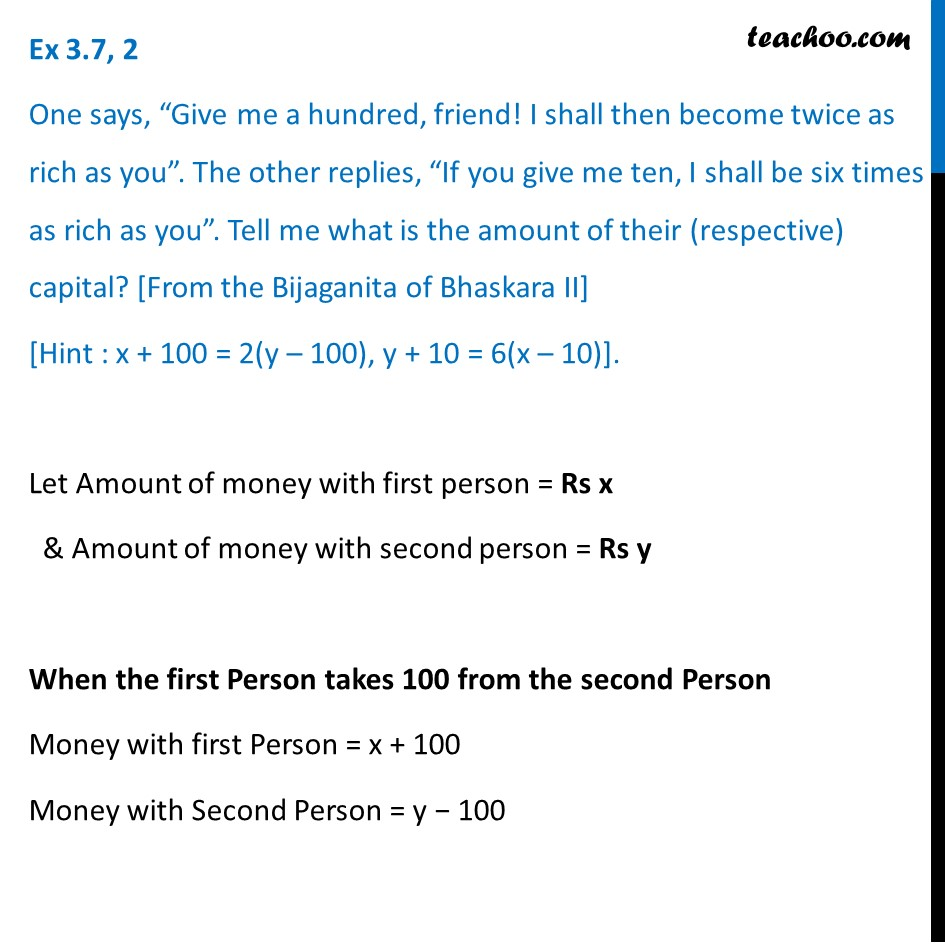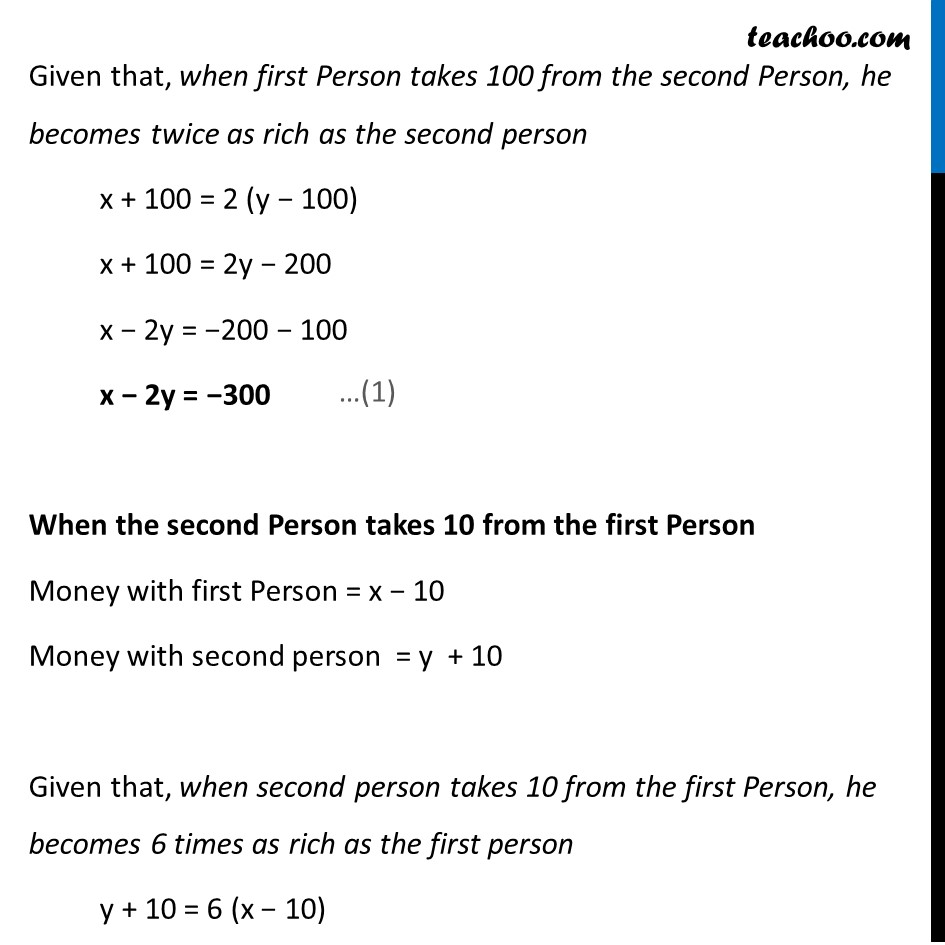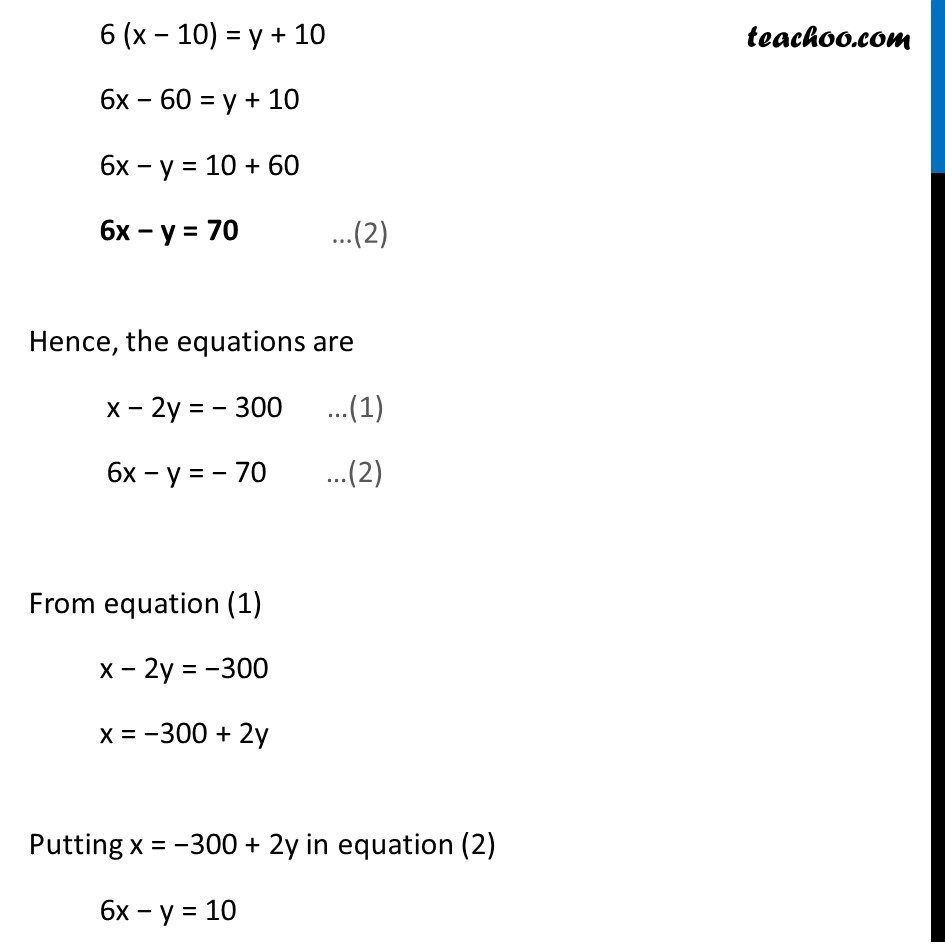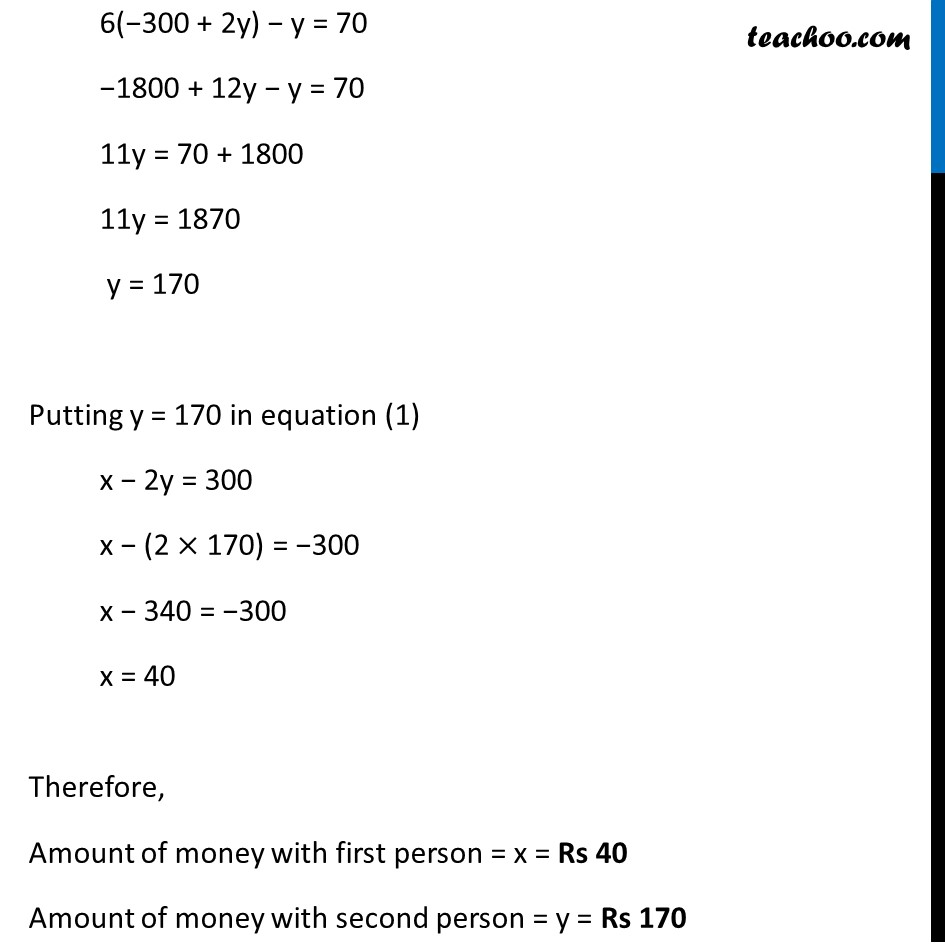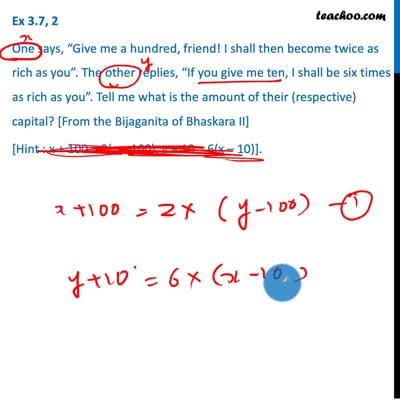This video is only available for Teachoo black users

Maths Crash Course - Live lectures + all videos + Real time Doubt solving!

### Transcript

Ex 3.7, 2 One says, “Give me a hundred, friend! I shall then become twice as rich as you”. The other replies, “If you give me ten, I shall be six times as rich as you”. Tell me what is the amount of their (respective) capital? [From the Bijaganita of Bhaskara II] [Hint : x + 100 = 2(y – 100), y + 10 = 6(x – 10)]. Let Amount of money with first person = Rs x & Amount of money with second person = Rs y When the first Person takes 100 from the second Person Money with first Person = x + 100 Money with Second Person = y − 100 Given that, when first Person takes 100 from the second Person, he becomes twice as rich as the second person x + 100 = 2 (y − 100) x + 100 = 2y − 200 x − 2y = −200 − 100 x − 2y = −300 When the second Person takes 10 from the first Person Money with first Person = x − 10 Money with second person = y + 10 Given that, when second person takes 10 from the first Person, he becomes 6 times as rich as the first person y + 10 = 6 (x − 10) 6 (x − 10) = y + 10 6x − 60 = y + 10 6x − y = 10 + 60 6x − y = 70 Hence, the equations are x − 2y = − 300 …(1) 6x − y = − 70 …(2) From equation (1) x − 2y = −300 x = −300 + 2y Putting x = −300 + 2y in equation (2) 6x − y = 10 6(−300 + 2y) − y = 70 −1800 + 12y − y = 70 11y = 70 + 1800 11y = 1870 y = 170 Putting y = 170 in equation (1) x − 2y = 300 x − (2 × 170) = −300 x − 340 = −300 x = 40 Therefore, Amount of money with first person = x = Rs 40 Amount of money with second person = y = Rs 170